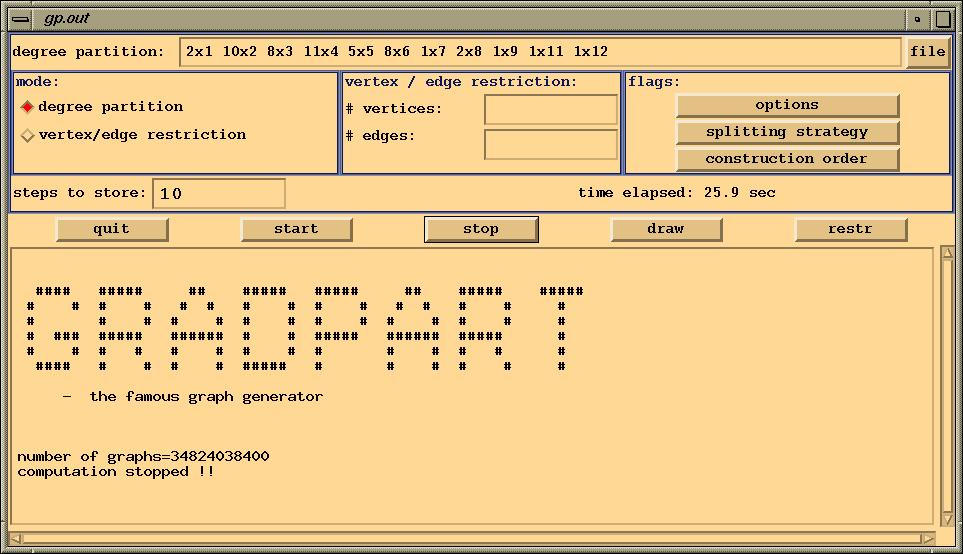GRADPART is a C++ - program mainly developed in my diploma-thesis. It works with a combination of homomorphism-principle and orderly generation. It computes all isomorphism-types of graphs with a given degree-sequence by recursively splitting the vertices of maximal degree ( until regular graphs are reached ), and gluing the pieces together to the whole graphs. If you want to know a bit more about the mathematical theory, then click here. There exists a graphical user-interface for Linux,SGI and Dec-Alpha written with Motif. You can get the package, by clicking here.

The mainwindow where you can choose the degree-partition looks like:In this example you can see a degree-partition with 50 vertices. Here we have 2 vertices of degree 1, 10 vertices of degree 2, 8 vertices of degree 3,... . Because of the use of the homomorphism-principle, during the generation we may obtain situations, where the operating group is trivial. So we get the possibility to describe large sets of pairwise non-isomorphic solutions implicitly. In this way in the shown example, we computed 34824038400 graphs in about 25 seconds and are also able to store these graphs with a very small amount of space.